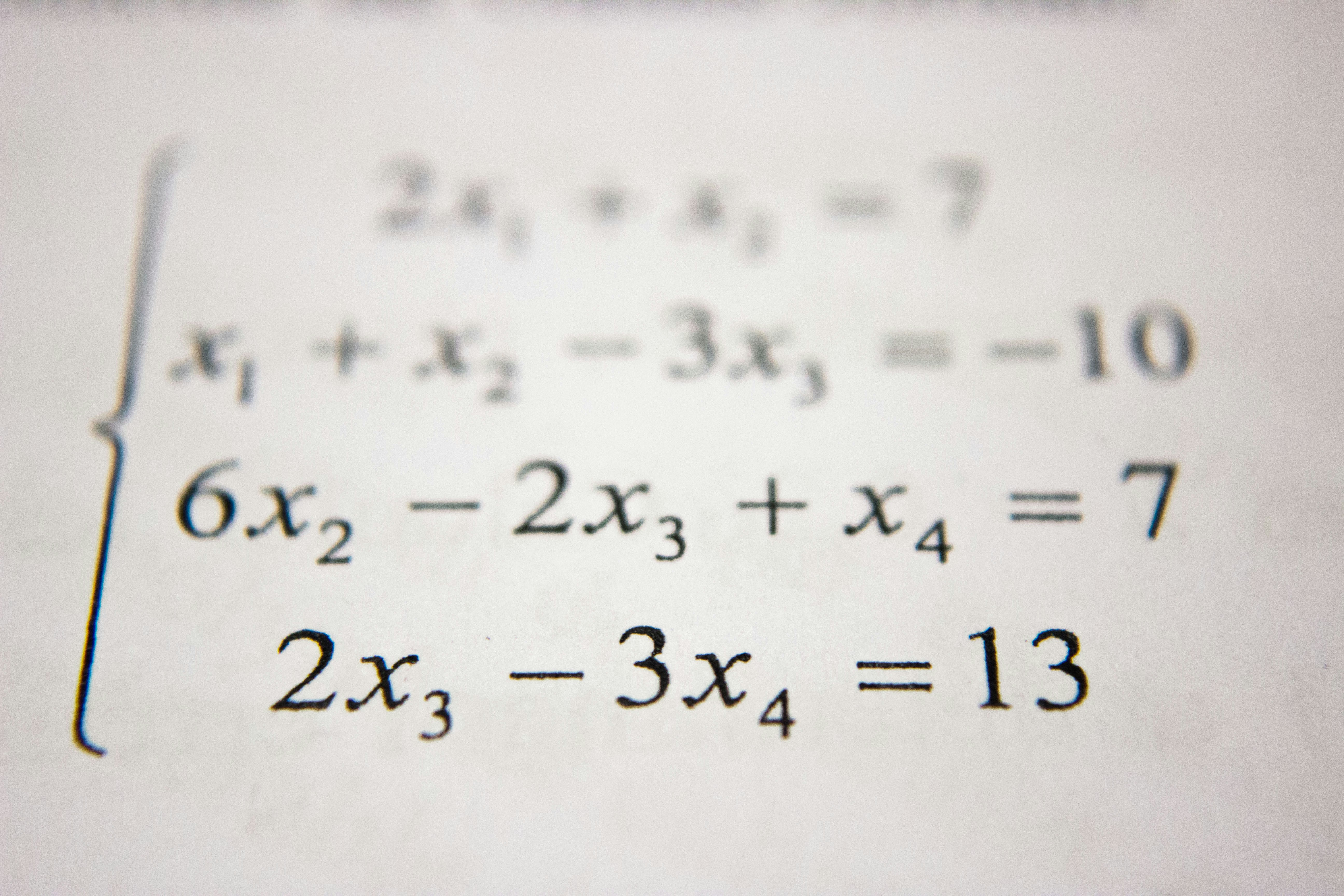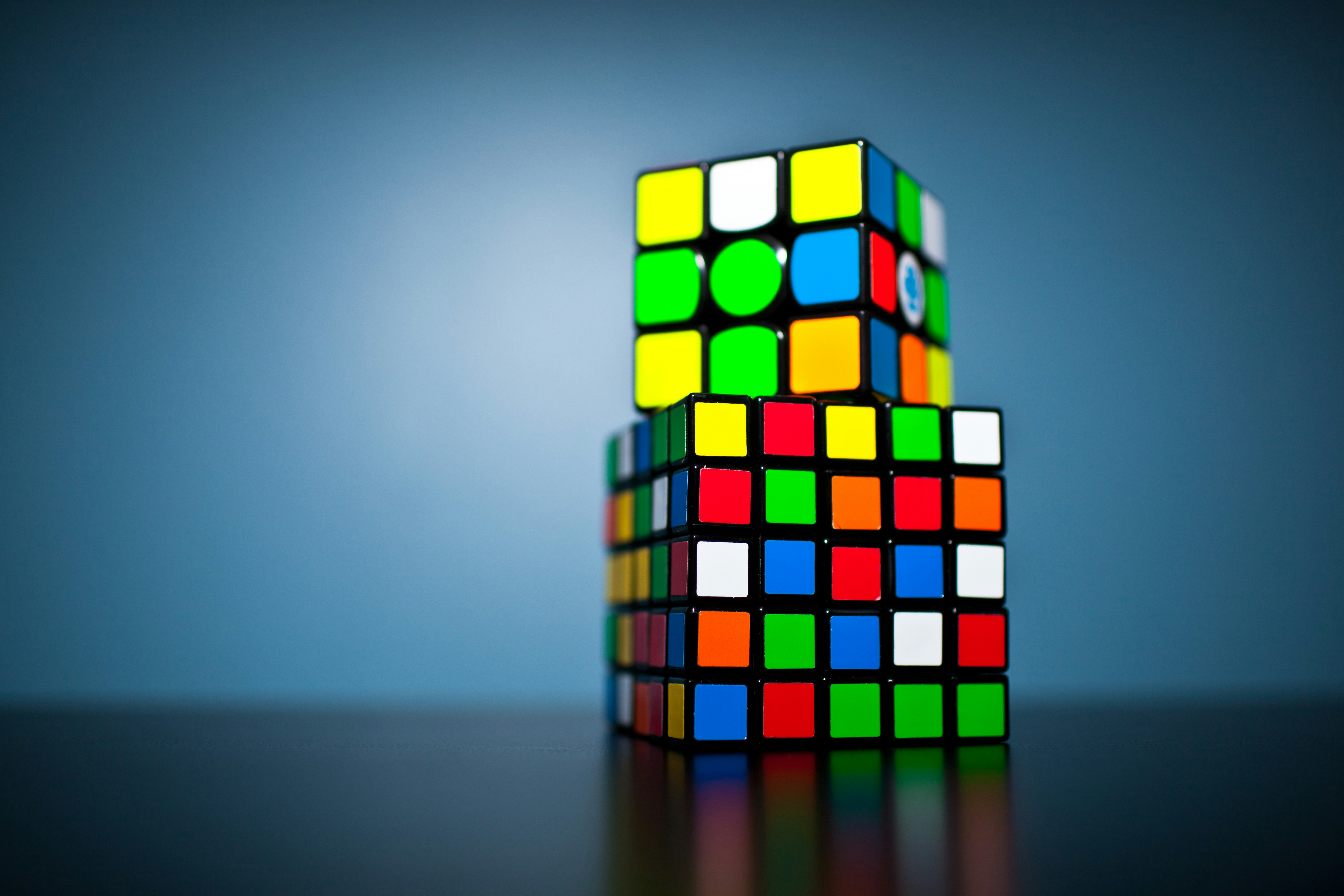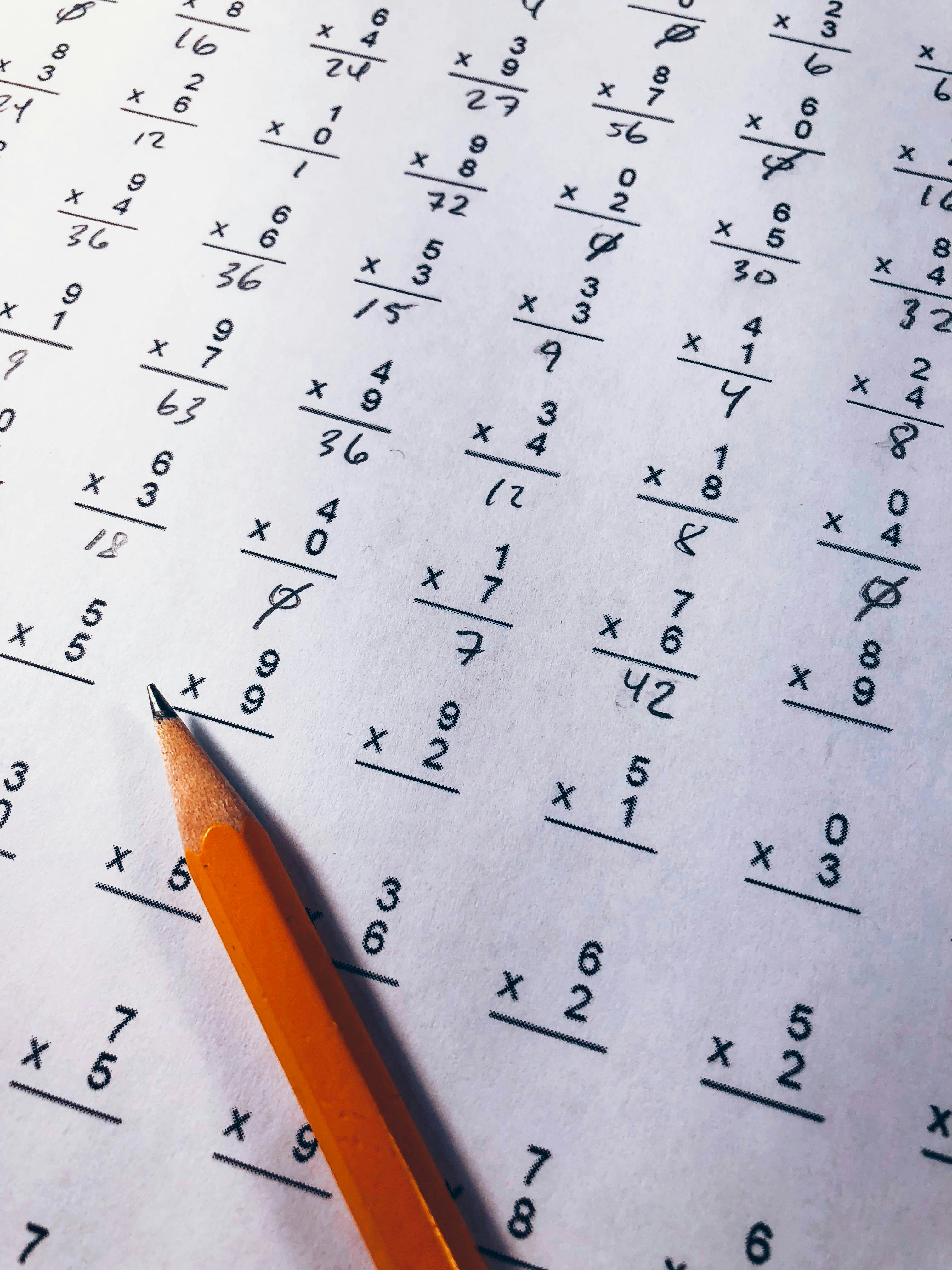# Mathematics

## A collection of 10 postsMathematics

## Half Derivatives and some Intuitive Fractional Calculus of Polynomials

As you may or may not know, polynomials are expressions that consists of only addition, subtraction, and multiplication operations.Mathematics

## Volume of an n-Dimensional Sphere

I remember, when I was an undergrad, at one of the beginner courses for physics, the teacher was lecturing about how to convert coordinates to and from Polar, Cylindrical, andMathematics

## Numerical Methods, Square Roots

When calculating square roots computers we can employ various techniques. IntroductionI remember in high school, I had this great math teacher teaching algebra. She taught that a we can findMathematics

## Error Functions, Stochastic Gradient Descent, & Backpropagation

oth the Stochastic Gradient Descent and Backpropagation are important concepts to understand when studying Neural Networks. Stochastic Gradient Descent optimizes the weights and Backpropagation finds the gradient of the error function quickly.Neural Networks

## Structure, Feedforward Neural Networks

A lot of times, Neural Networks are talked about in a purely conceptual way, leaving lea way for someone, who is trying to understand it's mechanics, room for misunderstandings. IntroHere,Mathematics

## Image Noise Reduction using SVD Decomposition

The greatest Mathematicians, as Archimedes, Newton, and Gauss, always united theory and applications in equal measure. - Felix KleinI remember, while taking a class in linear algebra, the professor, showingMathematics

## Padé Approximant & LU Decomposition, F# Implementation

A Padé Approximant is as the book by George A. Baker, Jr., "Essentials of Padé Approximants", the ratio of two polynomials created from the coefficients of the Taylor series of a function.Mathematics

## Monte Carlo Method, Integrals

IntroductionFor a while, while learning about Numerical Methods for integral, solutions of differential equation, and some other mathematical object, I've come across the Monte Carlo methods. It seemed like someMathematics

## Approximating the Gamma Function with the Gauss-Laguerre quadrature

Introduction In mathematics, when an exclamation mark, called a factorial, is used after a number it represents the multiplication of all the numbers from $0$ up to that number. ForMathematics

## Faster Matrix Multiplication, Strassen Algorithm

For a really long time it was thought that in terms of computational complexity the naive algorithm for the multiplication of matrices was the optimal one, wrong!